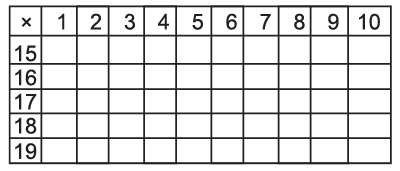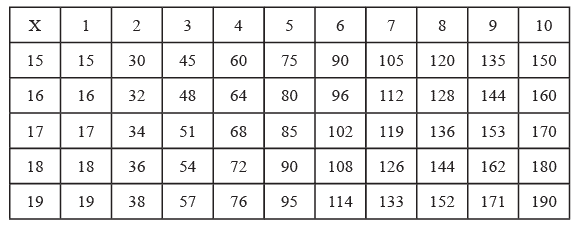Courses

# Worksheet Questions(Part - 2) - Knowing Our Number Class 6 Notes | EduRev

## Class 6 : Worksheet Questions(Part - 2) - Knowing Our Number Class 6 Notes | EduRev

The document Worksheet Questions(Part - 2) - Knowing Our Number Class 6 Notes | EduRev is a part of the Class 6 Course Mathematics (Maths) Class 6.
All you need of Class 6 at this link: Class 6

Ques 1: Fill up the blanks:
(a) Smallest 6-digit number in Indo Arabic Numeration System is __________.

(b) Largest 8-digit number in International Numeration System is
__________.
(c) Expanded notation of 2730145 is __________ .
(d) MDCL in Hindu-Arabic form is written as __________ .
(e) 707 in Roman Numerals can be written as __________ .
Ans: (a) One lakh
(b) Ninety nine million nine hundred ninety nine thousand nine hundred ninety nine.
(c) 2 × 10,00,000 + 7 × 1,00,000 + 3 × 10,000 + 0 × 1000 + 1 × 100 + 4 × 10 + 5
(d) 1650
(e) DCCVII

Ques 2: Using 2, 0, 4, 5 write largest and smallest 4-digit number (without repetition).

Ans: Largest number : 5420 Smallest number : 2045

Ques 3: Fill up the blanks using > or < signs:
(i) 2347 ________  2437 ________  2473 ________  2734 ________  2743

(ii) 50725 ________  50572 ________  50527 ________  50275 ________  50257
Ans. (i) 2347 < 2437 < 2473 < 2734 < 2743
(ii) 50725 > 50572 > 50527 > 50275 > 50257

Ques 4: Add 825432 and 543082 and write the sum according to Indo Arabic Numeration System.
Ans: Sum = 13,68,514 (Thirteen lakh sixty eight thousand five hundred and fourteen.)

Ques 5: Subtract 405235 from 995432 and write the difference according to International system of numeration.
Ans:
Difference = 590,197 (Five hundred ninety thousand one hundred ninety seven.)

Ques 6: If in a garden there are 4592 flowering plants and 3257 fruit trees then what's the total number of plants in the garden?
Ans:
Total number of plants = 7849

Ques 7: Evaluate : 81 [15 {7 – 2 (7 – 3)}]
Ans: –1215

Ques 8: A flask has 5 litres of lemonade. How many glasses, each of 200ml capacity, can it fill?
Ans: Number of glasses = 25

Ques 9: Estimate each of the following using general rule:
(i) 842 + 1245
(ii) 19,643 – 13,775
(iii) 2149 × 493
(iv) 5762 ÷ 287
(v) 439 + 8325 – 387
Ans: (i) 2,000
(ii) 6,000
(iii) 10,50,000
(iv) 20
(v) 8,000

Ques 10: Fill up the blanks:Ans:Offer running on EduRev: Apply code STAYHOME200 to get INR 200 off on our premium plan EduRev Infinity!

## Mathematics (Maths) Class 6

185 videos|229 docs|43 tests

,

,

,

,

,

,

,

,

,

,

,

,

,

,

,

,

,

,

,

,

,

;